GMAT Question of the Day: Daily via email | Daily via Instagram New to GMAT Club? Watch this Video

 It is currently 06 Aug 2020, 02:56### GMAT Club Daily Prep

#### Thank you for using the timer - this advanced tool can estimate your performance and suggest more practice questions. We have subscribed you to Daily Prep Questions via email.

Customized
for You

we will pick new questions that match your level based on your Timer History

Track

every week, we’ll send you an estimated GMAT score based on your performance

Practice
Pays

we will pick new questions that match your level based on your Timer History

#### Not interested in getting valuable practice questions and articles delivered to your email? No problem, unsubscribe here.# If O is the center of the circle with diameter AB and T is a tangency

Author Message
TAGS:

### Hide Tags

GMATH TeacherP
Status: GMATH founder
Joined: 12 Oct 2010
Posts: 938
If O is the center of the circle with diameter AB and T is a tangency  [#permalink]

### Show Tags

1
1000:00

Difficulty:95% (hard)

Question Stats:41% (02:49) correct59% (02:23) wrongbased on 39 sessions

### HideShow timer Statistics

GMATH practice exercise (Quant Class 7)If O is the center of the circle with diameter AB and T is a tangency point, what is the value of x?

(A) 10
(B) 15
(C) 18
(D) 20
(E) 22.5

_________________
Fabio Skilnik :: GMATH method creator (Math for the GMAT)
Our high-level "quant" preparation starts here: https://gmath.net
GMAT Club LegendV
Joined: 11 Sep 2015
Posts: 4999
GMAT 1: 770 Q49 V46
Re: If O is the center of the circle with diameter AB and T is a tangency  [#permalink]

### Show Tags

1
Top Contributor
1
fskilnik wrote:
GMATH practice exercise (Quant Class 7)If O is the center of the circle with diameter AB and T is a tangency point, what is the value of x?

(A) 10
(B) 15
(C) 18
(D) 20
(E) 22.5

Since angles in a triangle add to 180°, we know that the missing angle = (90 - 2x)°Aside: Notice that (90 - 2x)° + 90° + 2x° =180°

Since angles on a line add to 180°, we know that the missing angle = (90 + 2x)°Aside: Notice that (90 - 2x)° + (90° + 2x°) =180°

Next, draw a line from the center to the point of tangency. One of our circle properties tells us that this line is PERPENDICULAR to the tangent line.Now focus on ∆ATO (below)
Since OT and OA are radii, we know that OT = OA, which makes ∆ATO an ISOSCELES triangle.
This means the other angle is ALSO (90 - 2x)°Next, since OT is PERPENDICULAR to the tangent line, the remaining angle must be 2x°Aside: Notice that (90 - 2x)° + 2x° =90°

Finally, we'll focus on the green triangle.Since angles in a triangle add to 180°, we can write: x° + 2x° + (90 + 2x)° = 180°
Simplify: 5x° + 90° = 180°
Solve to get: x = 18

RELATED VIDEO FROM MY COURSE

_________________
If you enjoy my solutions, you'll love my GMAT prep course.GMATH TeacherP
Status: GMATH founder
Joined: 12 Oct 2010
Posts: 938
If O is the center of the circle with diameter AB and T is a tangency  [#permalink]

### Show Tags

fskilnik wrote:
GMATH practice exercise (Quant Class 7)If O is the center of the circle with diameter AB and T is a tangency point, what is the value of x?

(A) 10
(B) 15
(C) 18
(D) 20
(E) 22.5

Hi, Brent! Thank you for your nice contribution and beautiful drawings!Alternate approach: (Click in the figure to "zoom" it! All angles are measured in degrees.)

$$? = x$$

$$\Delta BTC\,\,:\,\,\,3x + 2x = 90\,\,\,\,\,\, \Rightarrow \,\,\,\,\,\,? = {{90} \over 5} = 18$$

We follow the notations and rationale taught in the GMATH method.

Regards,
Fabio.
_________________
Fabio Skilnik :: GMATH method creator (Math for the GMAT)
Our high-level "quant" preparation starts here: https://gmath.net
DirectorP
Joined: 04 Aug 2010
Posts: 684
Schools: Dartmouth College
Re: If O is the center of the circle with diameter AB and T is a tangency  [#permalink]

### Show Tags

fskilnik wrote:
GMATH practice exercise (Quant Class 7)If O is the center of the circle with diameter AB and T is a tangency point, what is the value of x?

(A) 10
(B) 15
(C) 18
(D) 20
(E) 22.5

A radius drawn to a tangent point yields a RIGHT ANGLE: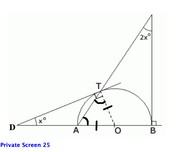In the figure above:
Radius OT forms a right angle at tangent point T.
OA and OT are radii and thus are equal.
Since OA=OT, opposite angles OAT and ATO are equal.

We can PLUG IN THE ANSWERS, which represent the value of x.
From left to right, option D yields the following 3 triangles: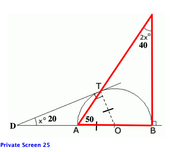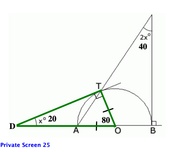In this case, the resulting angle sum for the green triangle is TOO BIG:
20+80+90 = 190.
Eliminate D.

From left to right, option B yields the following 3 triangles: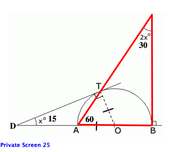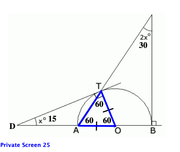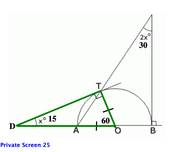In this case, the resulting angle sum for the green triangle is TOO SMALL:
15+60+90 = 165.
Eliminate B.

Since D yields a sum that is TOO BIG, while B yields a sum that is TOO SMALL, the correct answer must be BETWEEN B AND D.

.
_________________
GMAT and GRE Tutor
New York, NY

Available for tutoring in NYC and long-distance.
Non-Human UserJoined: 09 Sep 2013
Posts: 15605
Re: If O is the center of the circle with diameter AB and T is a tangency  [#permalink]

### Show Tags

Hello from the GMAT Club BumpBot!

Thanks to another GMAT Club member, I have just discovered this valuable topic, yet it had no discussion for over a year. I am now bumping it up - doing my job. I think you may find it valuable (esp those replies with Kudos).

Want to see all other topics I dig out? Follow me (click follow button on profile). You will receive a summary of all topics I bump in your profile area as well as via email.
_________________Re: If O is the center of the circle with diameter AB and T is a tangency   [#permalink] 01 Apr 2020, 17:33

# If O is the center of the circle with diameter AB and T is a tangency